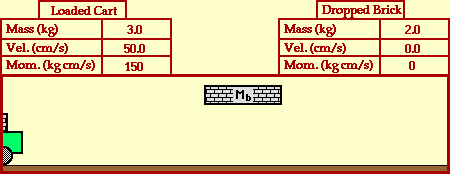## The Cart and The Brick

### Part A

Collisions between objects are governed by laws of momentum and energy. When a collision occurs in an isolated system, the total momentum of the system of objects is conserved. Provided that there are no net external forces acting upon the objects, the momentum of all objects before the collision equals the momentum of all objects after the collision. If there are only two objects involved in the collision, then the momentum lost by one object equals the momentum gained by the other object.

The animation below portrays the collision between a 3.0-kg loaded cart and a 2-kg dropped brick. It will be assumed that there are no net external forces acting upon the two objects involved in the collision. The only net force acting upon the two objects (loaded cart and dropped brick) are internal forces - the force of friction between the loaded cart and the dropped brick. The before- and after-collision velocities and momentum are shown in the data tables.In the collision between the loaded cart and the dropped brick, total system momentum is conserved. Before the collision, the momentum of the loaded cart is 150 kg*cm/s and the momentum of the dropped brick is 0 kg*cm/s; the total system momentum is 150 kg*cm/s. After the collision, the momentum of the loaded cart is 90.0 kg*cm/s and the momentum of the dropped brick is 60.0 kg*cm/s; the total system momentum is 150 kg*cm/s. The momentum of the loaded cart-dropped brick system is conserved. The momentum lost by the loaded cart (60 kg*cm/s) is gained by the dropped brick.

For more information on physical descriptions of motion, visit The Physics Classroom Tutorial. Detailed information is available there on the following topics:

Momentum

Momentum Conservation Principle

Isolated Systems

Momentum Conservation in Collisions